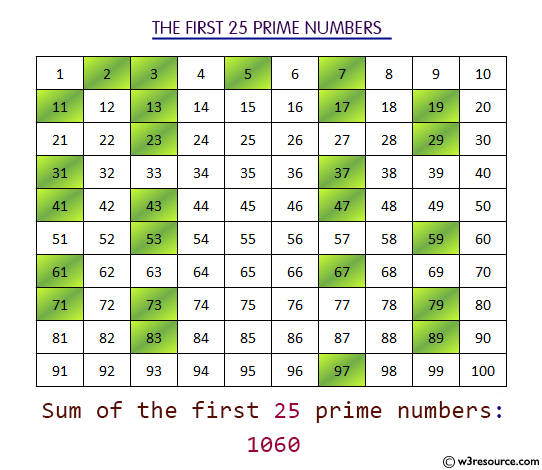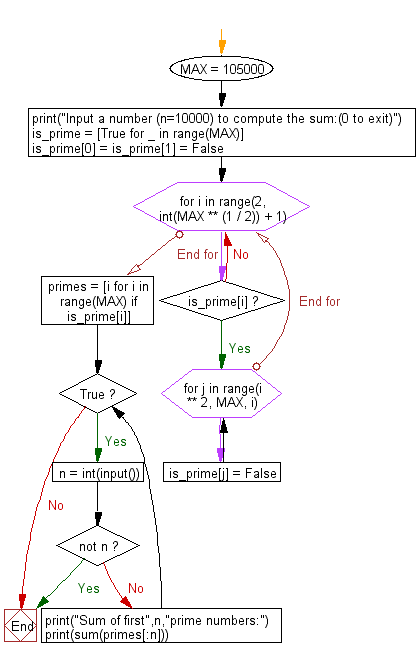﻿ Python: Compute the sum of first n given prime numbers - w3resource# Python: Compute the sum of first n given prime numbers

## Python Basic - 1: Exercise-52 with Solution

Write a Python program to compute the sum of first n given prime numbers.

Input:
n ( n ≤ 10000). Input 0 to exit the program.
Input a number (n≤10000) to compute the sum:(0 to exit)
25
Sum of first 25 prime numbers:
1060

Pictorial Presentation:Sample Solution:

Python Code:

``````MAX = 105000
print("Input a number (n≤10000) to compute the sum:(0 to exit)")
is_prime = [True for _ in range(MAX)]
is_prime = is_prime = False
for i in range(2, int(MAX ** (1 / 2)) + 1):
if is_prime[i]:
for j in range(i ** 2, MAX, i):
is_prime[j] = False
primes = [i for i in range(MAX) if is_prime[i]]
while True:
n = int(input())
if not n:
break
print("Sum of first",n,"prime numbers:")
print(sum(primes[:n]))
``````

Sample Output:

```Input a number (n≤10000) to compute the sum:(0 to exit)
25
Sum of first 25 prime numbers:
1060
```

Flowchart:Python Code Editor:

Have another way to solve this solution? Contribute your code (and comments) through Disqus.

What is the difficulty level of this exercise?

Test your Programming skills with w3resource's quiz.

﻿

## Python: Tips of the Day

What is the difference between Python's list methods append and extend?

append: Appends object at the end.

```x = [1, 2, 3]
x.append([4, 5])
print (x)
```

Output:

```[1, 2, 3, [4, 5]]
```

extend: Extends list by appending elements from the iterable.

```x = [1, 2, 3]
x.extend([4, 5])
print (x)
```

Output:

```[1, 2, 3, 4, 5]
```

Ref: https://bit.ly/2AZ6ZFq